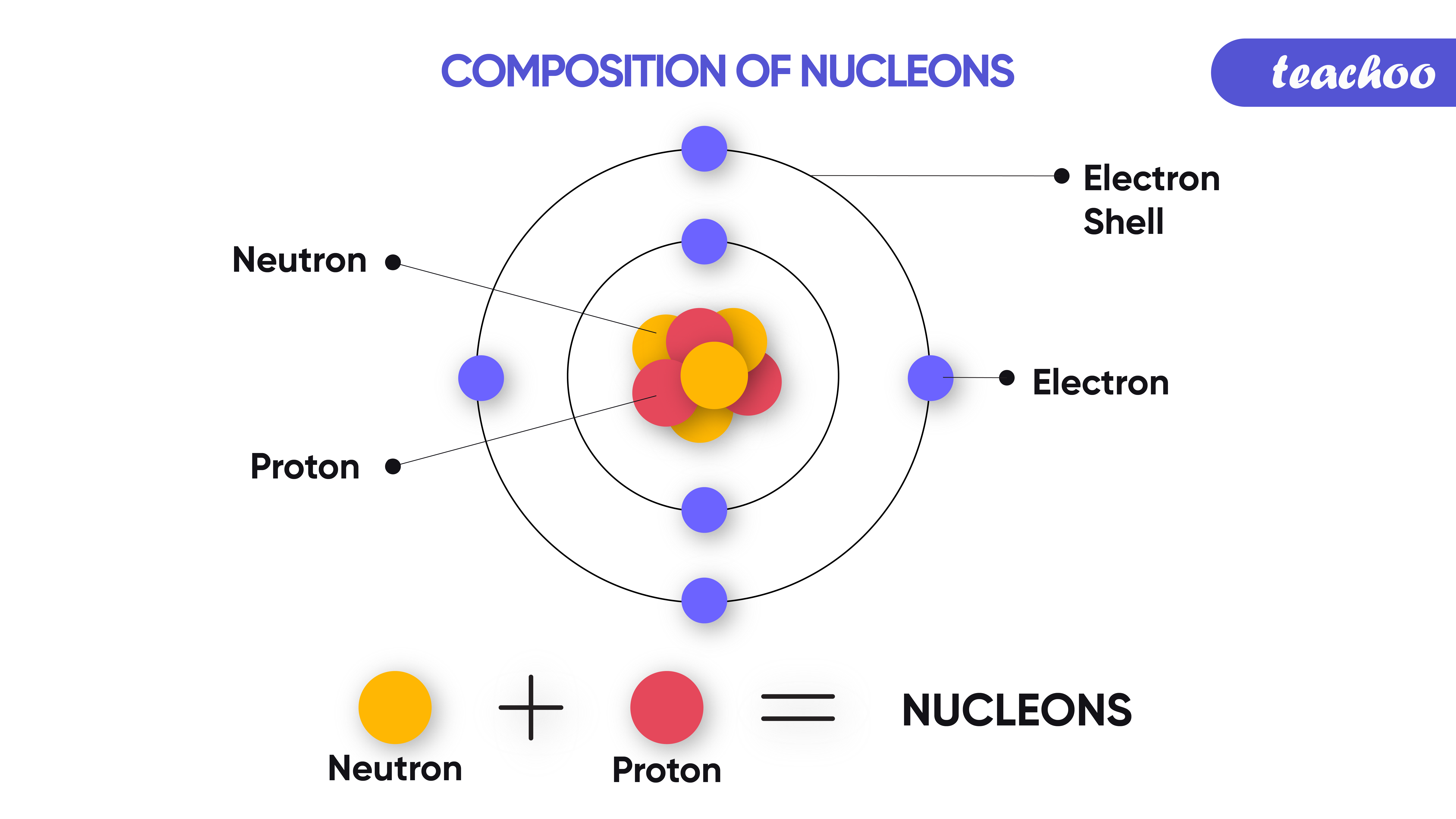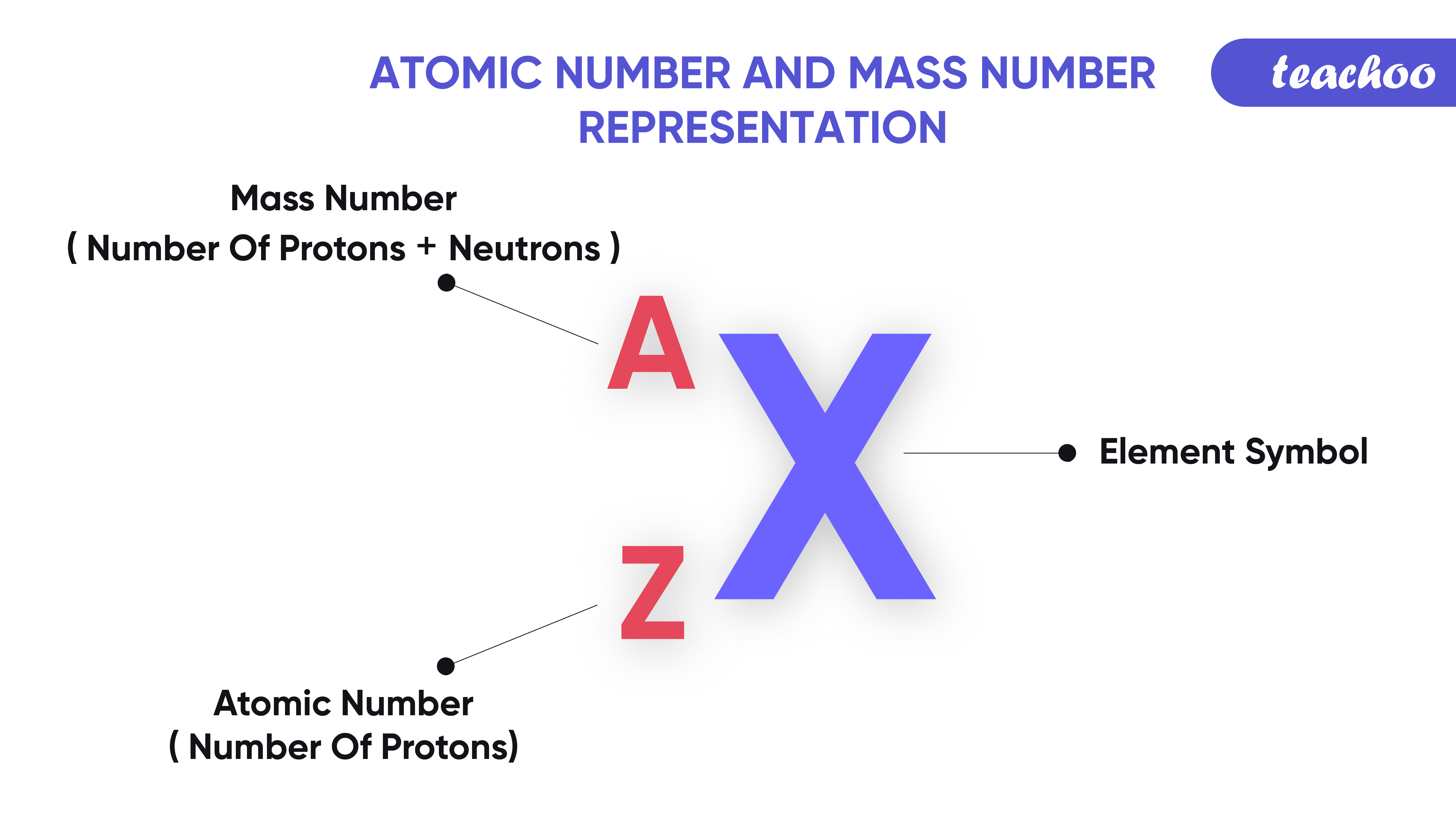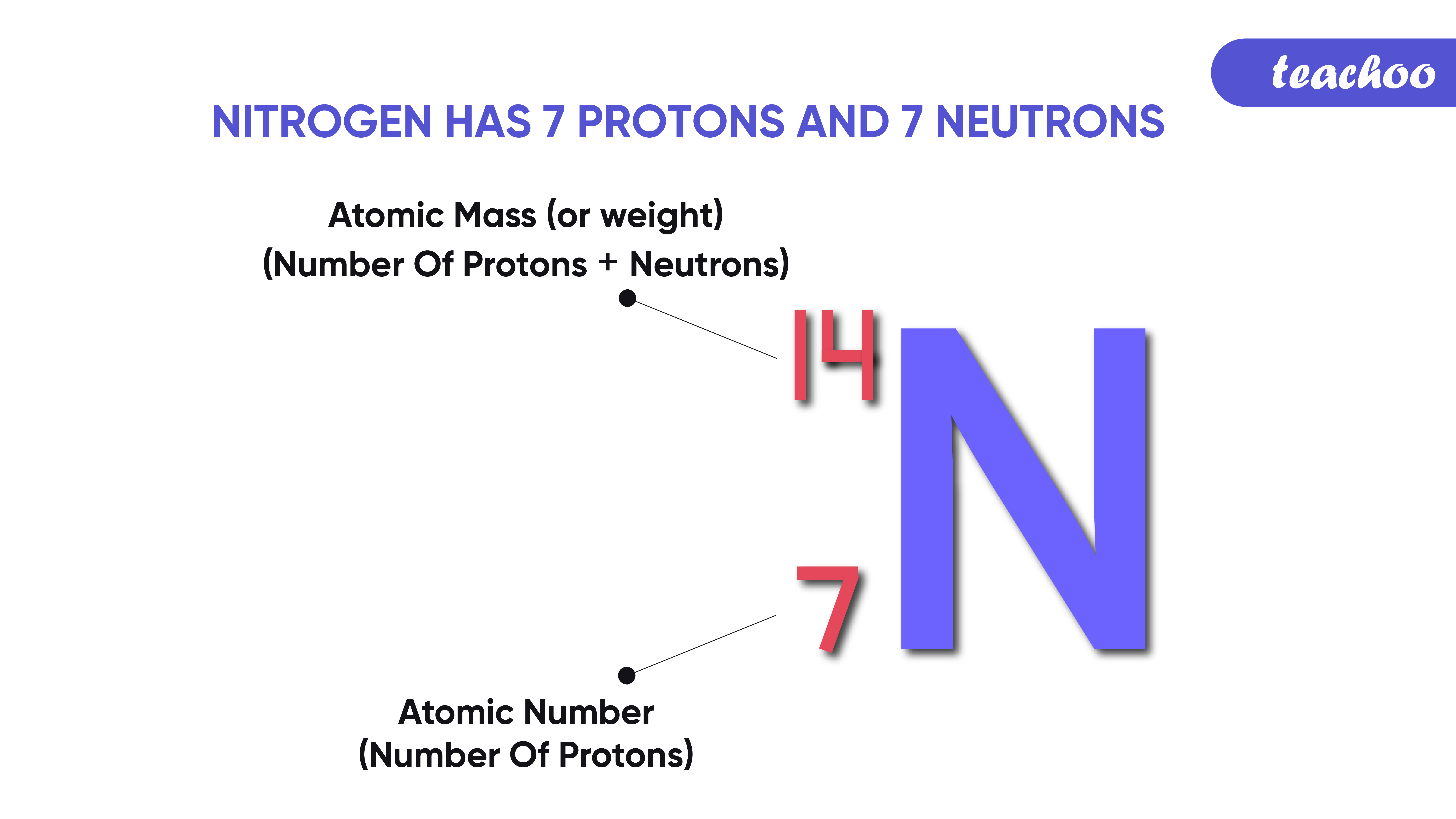## What are Nucleons?

• We know that every atom contains protons, neutrons and electrons
• Out of this, Protons and Neutrons are present in the nucleus of an atom.
• They are together called Nucleons .

## What is an atomic number?

• It is the number of protons present in the nucleus of an atom .
• It is denoted by Z .## Example - 1

• For Hydrogen, Atomic number (Z) = 1
• It means that in a hydrogen atom, there are 1 proton present in the nucleus.

## Example - 2

• For Carbon, Atomic Number (Z) = 6

It means that in a carbon atom, there are 6 protons in the nucleus.

## What is Mass Number?

• Mass number is the total number of protons and neutrons present in the nucleus of an atom.
• It is denoted by A .

## Example - 1

• Carbon atom has 6 protons and 6 neutrons
• So, Mass number is 6 + 6 = 12
• Mass number (A) = 12

## Example - 2

• An aluminium atom has 13 protons and 14 neutrons
• So, Mass number = 13 + 14 = 27
• Mass number (A)  = 27

## How is Atomic and Mass Number Represented?

• Atomic Number is represented as Subscript
• Mass Number is represented as Superscript## Example

• Nitrogen has 7 Protons and 7 Neutrons
• Hence Atomic Number = Z = No of Protons = 7

Mass Number = A = Number of Protons + Number of Neutrons = 7 + 7 = 14Examples -

In text Question -

NCERT Back Exercise - Q8 (i) (ii), Q19

1. Class 9
2. Chapter 4 Class 9 - Structure of Atom (Term 2)
3. Concepts

Concepts

Class 9
Chapter 4 Class 9 - Structure of Atom (Term 2)